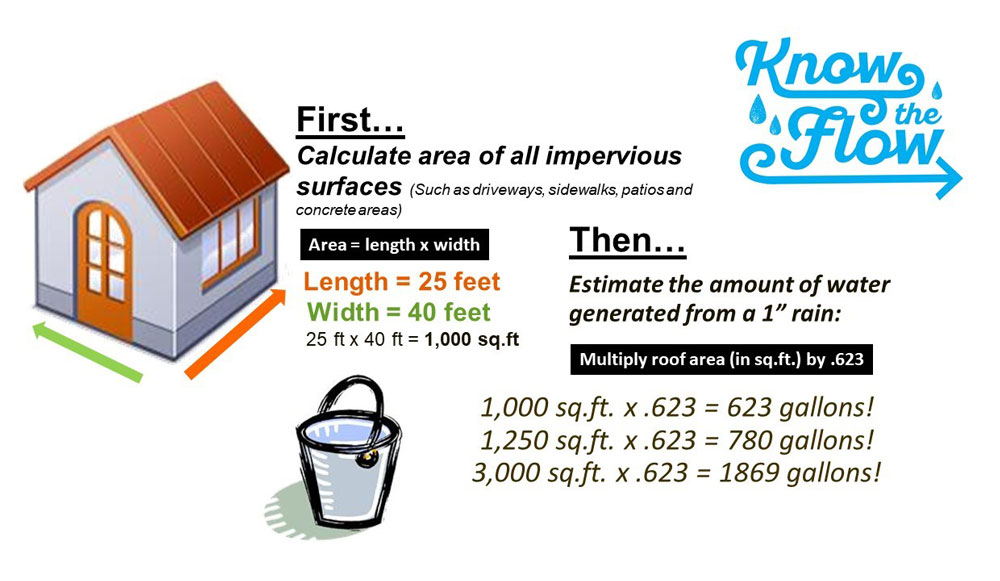How do I calculate stormwater runoff on my property?

# Step 3: Calculate the Runoff From Your Property (Determined by Best Management Practice)

This step is optional depending on your selected best management practice.

## How do I calculate stormwater runoff?

First, calculate the square foot of all hard, impervious surfaces such as driveways, sidewalks, and other roofed or concrete areas that do not allow water to soak in by measuring in feet and the multiplying length times width.

Area = length x width

Then, to determine the amount of runoff in gallons, multiply the total area in square feet by .623.

### Example Calculation

The impervious surfaces equaled 25 ft in length and 40 ft in width. So that would be 25 ft x 40 ft = 1,000 sq.ft

Then we multiple the area by .623. So that would be 1,000 sq.ft x .623 = 623 gallons!Top